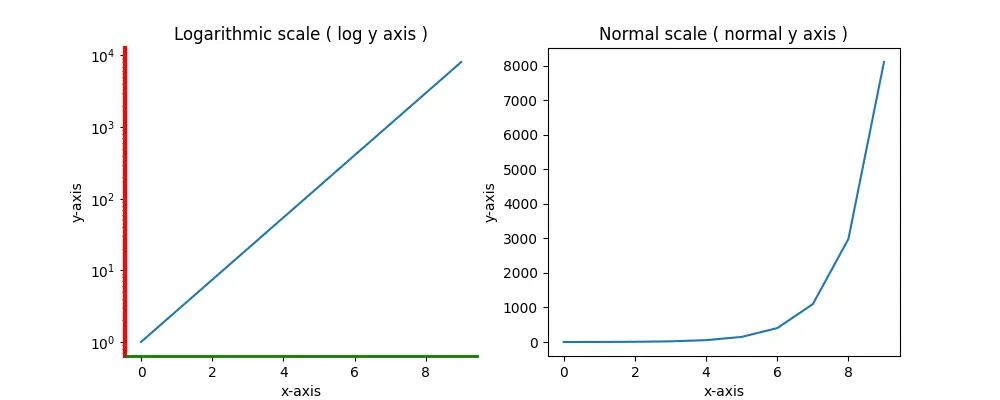# How To Set Matplotlib Axis Format

In a function graph, sometimes the independent variable X and the dependent variable Y correspond exponentially, so the scale of the coordinate axis needs to be set to a logarithmic scale. This article will tell you how to implement it in Matplotlib by resetting the axis format with examples.

### 1. How To Set Matplotlib Axis Format.

1. Matplotlib uses the xscale or yscale attribute of the axes object to format the axes.
```# set the first axes y axis format to 'log'.
axes_left.set_yscale('log')```
2. You can use the axes object’s attribute spines ( a python dictionary object ) to set the figure’s border’s style such as color, linewidth, etc.
```# set the y-axis color and line width.
axes_left.spines['left'].set_color('red')
axes_left.spines['left'].set_linewidth(3)```
3. In the below example, there are 2 figures. The left figure’s axis has been modified, and the right figure’s axis is normal.
```import matplotlib.pyplot as plt

import numpy as np

def matplotlib_set_axis_format():

# divide the figure canvas to 1 row and 2 columns.
fig, axes = plt.subplots(1, 2, figsize=(10,4))

# get the first axes object.
axes_left = axes

# get the second axes object.
axes_right = axes

# calculate x array.
x = np.arange(0, 10)

# calculate the y values.
y = np.exp(x)

axes_left.set_title('Logarithmic scale ( log y axis )')
axes_left.set_xlabel('x-axis')
axes_left.set_ylabel('y-axis')

# set the first axes y axis format to 'log'.
axes_left.set_yscale('log')

# set the y-axis color and line width.
axes_left.spines['left'].set_color('red')
axes_left.spines['left'].set_linewidth(3)

# set the x-axis color and line width.
axes_left.spines['bottom'].set_color('green')
axes_left.spines['bottom'].set_linewidth(2)

# hide the right and top border.
axes_left.spines['right'].set_color(None)
axes_left.spines['top'].set_color(None)

# call the plot function to draw the figure.
axes_left.plot(x,y)

axes_right.set_title('Normal scale ( normal y axis )')
axes_right.set_xlabel('x-axis')
axes_right.set_ylabel('y-axis')
axes_right.plot(x,y)

# show the figure.
plt.show()

if __name__ == '__main__':

matplotlib_set_axis_format()```
4. When you run the above example, it will create the below picture.This site uses Akismet to reduce spam. Learn how your comment data is processed.